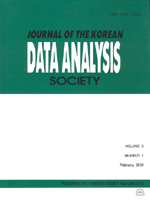상세검색
최근 검색어 전체 삭제
다국어입력
즐겨찾기0KCI등재 학술저널

# A Study on the Nonparametric Tests for the Two-Sample Problem

• 등재여부 : KCI등재
• 2010.10
• 2431 - 2442 (12 pages)

In this paper, we consider nonparametric test procedures based on a group of quantile test statistics. Since we do not assume any specific model, this new nonparametric tests may be useful when the underlying distributions are completely unknown. We consider the quadratic form of statistic for the general alternatives and derive the chi-square distribution as the limiting distribution for the large sample case. Then we illustrate our procedure with an example and compare the proposed tests with the individual quantile tests by obtaining empirical powers through simulation study by applying the permutation principle. Also we comment on the related discussions to this testing procedure as concluding remarks.

1. Introduction

2. New nonparametric tests

3. An example, simulation study and some concluding remarks

References